individual_assignment_tensorflow_playground
Updated automatically every 5 minutes

An introduction to building and training neural networks

This week's individual assignment will make use of the interactive website https://playground.tensorflow.org/. This website allows you to tinker with a (deep) neural network and see how different settings and parameters change its mechanisms and outputs.

## Introduction

First of all, go to https://playground.tensorflow.org. This should load a page with a pre-configured (deep) neural network, which you can interact with. Before you start messing around with the network, read the text below it.

## Setting up a simple network

Let's start with setting up a very simple, "shallow" neural network. Change the number of hidden layers from 2 to 0. Under DATA, select the dataset with the two clearly separated clusters (see image below). Set the activation to "Linear". Leave the rest as-is.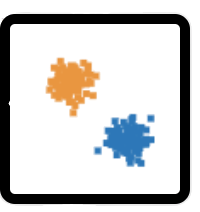You just built a "shallow" neural network for a classification problem with two classes: orange (0) and blue (1). This network will try to use two variables, x1 and x2 (the input, or independent variables), to classify the different observations (dots in the rightmost graph) as their correct class (the dependent variable). In other words, it will try to classify orange dots as orange (class 0) and blue dots as blue (class 1). For those with a statistics background: this is actually very similar to the structure of a vanilla logistic regression model!

### Question 1 (1 point)

Can you think of an example of what x1 and x2 (the input variables) and "orange" and "blue" (the classes) could represent?

## Weights

Right now, the network is untrained! That is, it is configured with random weights (sometimes called "parameters"). These weights will be multiplied and summed with the input variables (and rescaled using the activation function, which we'll discuss in detail later, so you can ignore this for now). This multiplication-and-summing process of the variables and weights is an integral component of neural networks. As an analogy, try to think of training as baking a cake, in which the input variables represent the ingredients and the weights represent the quantities of the ingredients.

### Question 2 (1 point)

Alright, for an easy question to test whether you're still awake: how many weights does this particular network have?

## Decision surface and loss

Because the network starts off with random weights, its initial performance will most likely be quite bad. You can see this in the rightmost plot, which visualizes the "decision surface", which colors the space according to the class it would predict an observation. So, dots in blue regions will be classified as blue and dots in orange regions will be classified as orange. The saturation of the color represents the confidence of the decision (more saturation indicates a higher confidence).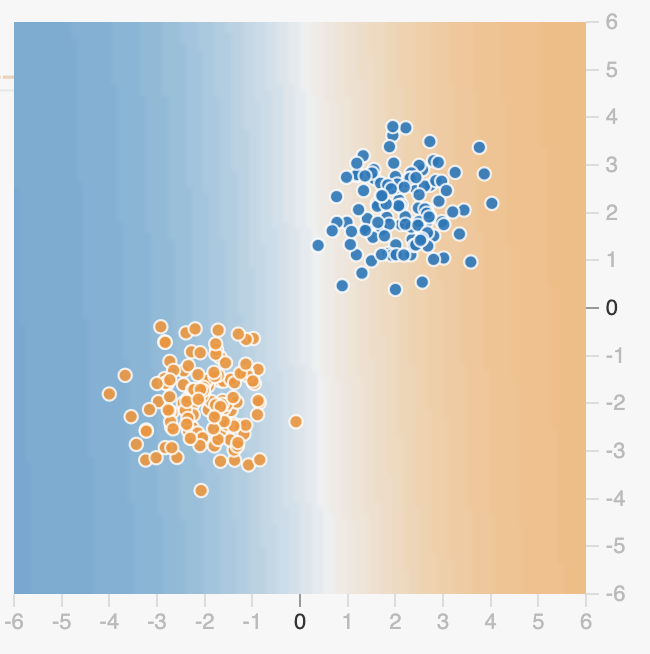How badly the network is currently classifying the observation is summarized as its loss. Loss can be computed in different ways (using different loss functions), but in general a higher loss means a worse performing network. The current loss of the (untrained) network is displayed underneath OUTPUT. This is reported separately for the "train" and "test" set. The train and test set are often drawn from the same dataset by splitting the full dataset into two partitions (e.g., 50% train, 50% test). The train set is subsequently used to, well, train the network and the test set is used to evaluate how well the network is doing.

By default, only the train set is shown in the rightmost graph; to visualize the test set, select the "Show test data" checkbox. For now, you can ignore this distinction between train and test set; we'll discuss this in more detail later.

### Question 3 (1 point)

Instead of letting the network train itself, we can try to "train" it ourselves by manually setting the weights! You can set the weights yourself by clicking the dashed lines (representing the weights) and editing the current (random) value.

Try out different values for the weights (or try to deduce which values the weights should have). Which values lead to a good classification performance (i.e., low loss)? Write them down below (this does not have to be very precise; there are many possible correct answers).

## Training

Now, let's train the network! In the context of neural networks, training refers to the process of (iteratively) adjusting the network's weights based on the loss such that, over time, the loss becomes as small as possible.

There are a couple of options, or settings, that influence the training procedure. One is the specific loss function (sometimes called "objective function") used; this cannot be changed on this website. Another important one is "batch size", which represents the number of observations that will be passed through the network before computing the loss and updating the weights based on this loss. This might sound weird to you, as many of the more traditional statistical models you are familiar with just use all data available. However, many (deep) neural networks are trained on massive datasets, which may include millions of observations (such as imagenet). Passing such amounts of data to a network at once will crash any computer! Therefore, networks are often iteratively trained on batches of observations with a particular batch size. (In our example, however, we're dealing with relatively little data, so it is not strictly necessary to train the network in batches.)

Other important training settings are the learning rate and amount of regularization, which we discuss later.

### Question 4 (1 point)

Click on the "play" button to start training the network! After a couple of seconds, press it again to pause the training. You should see that the counter under the word "epoch" has increased. Epochs are the number times the full dataset (i.e., all observations) has passed through the network.

Suppose that our dataset has 500 examples and we set the batch size to 25. After 200 epochs, how many times has our network updated its weights?

## Convergence

You can see that the network's weights change relatively little after a 100 epochs or so. This is nicely visualized as a graph next to the loss values (with loss on the y-axis and epochs on the x-axis; see below). When a network stops updating its weights over time, it is said that the network has converged.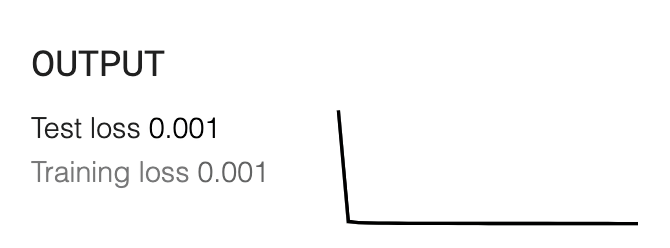## Nonlinear problems

So far, we have dealt with a relatively easy problem: classifying observations drawn from two distinct, relatively noiseless, clusters. Importantly, this represented a "linearly separable" problem: accurate classification could be achieved by drawing a straight (i.e., non-curved) line. This type of problem can often be solved by relatively simple models, including models from traditional statistics (such as logistic regression and linear discriminant analysis). This type of problem is not where neural networks shine. So let's subject our neural network to a more challenging problem.

Change the dataset to the one with the orange ring with the blue cluster inside (see below); note that this is a nonlinear problem (i.e., its observations cannot be accurately predicted by drawing a straight line). Then, using the settings we used up till now, start training the network.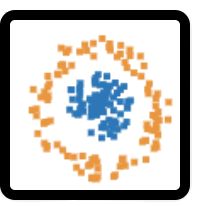As opposed to the previous dataset, you should see that our network is doing terribly! The loss stays around 0.5 and doesn't decrease... The reason for this failure is that our network is completely linear and thus cannot solve a nonlinear problem! Any network that only involves only the multiplication-and-summing of values and weights (in combination with a linear activation function) can only solve linearly separable problems...

## Let's go deeper!

So, how can we make our network nonlinear? There are different ways to do this. One popular way is to add hidden layers. But before we'll discuss hidden layers, can you think of a way to add nonlinearity (in a way that solves our current problem) that doesn't involve adding hidden layers? Try it on the website! This is not graded.

## Hidden layers

Hidden layers are like an intermediary step between the input and the output. The nodes (or units/neurons/variables) of the hidden layers are the result of a separate multiplication+sum+activation step of the previous layer (in this case, the input layer).

For now, add a single hidden layer with three neurons. Note the way the input layer is "wired" to the hidden layer: each input unit has a connection with each hidden unit. (This is the reason why people sometimes call these types of networks "fully-connected networks".) Also, note that the hidden layer is now directly connected to the output. In total, this results in a network with 9 weights.

### Question 5 (1 point)

Now, for a slightly harder question: suppose we have a network with 3 input variables and two hidden layers with each five hidden units; how many weights does this network have?

## Activation functions

Start training this network! Disappointingly, you should note that it doesn't seem to be able to solve this nonlinear problem... The reason for this, as we previously noted, is that this network only involves linear operations (multiplication-and-summing) and any network that only involves linear operations won't ever be able to solve a nonlinear problem. See for yourself: change your network to 6 hidden layers with each 8 neurons and start training again -- it still won't solve this nonlinear problem! (Change it back to a single hidden layer with 3 neurons when you're done.)

So, hidden layers are not enough... The solution has in fact to do with the activation function. As discussed, the activation function can be thought of as a function that rescales the data: it receives the result of the multiplication-and-summing operation (a single value) and outputs a rescaled version of that value. The way the data is rescaled depends on the activation function, but what they have in common is that they are often nonlinear functions (except for, as you would've guessed, the linear activation function). As such, we can use a nonlinear activation function to "inject" nonlinearity in our network!

Set the activation function to "tanh" and re-train the network!

## Hidden layer representations

Yeah! After about 100 epochs, you should see that the network accurately classifies most observations! In other words, it has solved this particular nonlinear classification problem! The way it does this is by combining the (slightly) nonlinear units, or "representations", from the hidden layer to create a nonlinear decision surface. To zoom in on the representations from the hidden layer, hover over a particular hidden unit, which will show the decision surface of that particular neuron in the rightmost graph (i.e., it shows the decision surface of the network if the network was only allowed to use that neuron). By doing this, you can see that the final decision surface is the result of the combination of the hidden layer decision surfaces!

## Overfitting

So far, we have used a rather "idealized", mostly noiseless dataset. Real data is often not so neat. We can simulate this by setting the noise level to 50 (drag the slider all the way to the right). You'll still notice the orange circle + blue cluster inside it, but it's more noisy. Also, let's pretend that we actually have less data by reducing the train set; do this by setting the "Ratio of training to test data" to 30%.

Now, start training the network again. Note that it may take longer (i.e., more epochs) to create a reasonable decision surface like before, because the data is noisier. Also, it is likely that you see that the loss of the train and test set data seem to diverge over time: the loss of the train set is decreasing (as expected) while the loss of the test set is increasing... (If this doesn't happen, try regenerating the dataset and restarting the training.) What is happening here?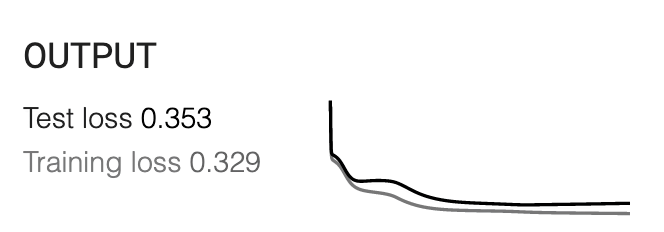The answer is overfitting: the divergence of accuracy of the model on the train set vs. accuracy on the test set, which happens more often in scenarios with relatively little data, a lot of noise, and/or when using very flexible and powerful models (e.g., neural networks with lots of layers and units per layer).

### Question 6 (1 point)

Explain in your own words what you think causes overfitting. (Answering having too little data/too much noise/too flexible model is not correct.)

## Regularization

There are different ways to counter overfitting. For example, you could try to get better measurements (less noise in your data), more data, or using a less flexible/powerful model. Another often used technique is regularization.  This technique tries to balance the learning process by imposing constraints on the weights (usually by limiting how large they can become; e.g., "L1" or "L2" regularization) or even randomly removing hidden units from the network ("dropout" regularization). Often, when the amount of regularization is chosen appropriately (which is sometimes a matter of trying out different settings), this leads to a better predictive generalization of the model.

Set the regularization to "L2" and the "Regularization rate" to 0.03 and re-train the network. You should see that the difference between the train and test set loss should become much smaller (or even invert — a phenomenon called "underfitting", suggesting a regularization rate that may be too high).

## Hyperparameter tuning

Alright, now that you know the basics of training neural networks, let's try an even more difficult classification problem! Change the dataset to the two intertwined "swirls" (see below), but set the noise level back to 0 (set the train set ratio to 50%). If you train this network, you'll see that our current neural network with a single hidden layer cannot really solve this problem... Because this is a harder (nonlinear) problem than the previous one, you'll have to make the network more complex!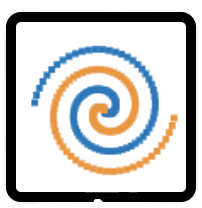Go nuts! Try adding extra layers, extra input variables, more units per hidden layer, another activation function, a lower/higher learning rate, etc. Note that you may have to add stronger regularization if you increase the complexity of the model. You'll see that it's quite hard to find the "optimum" set of settings (sometimes called "hyperparameters")!

Note down your best score (i.e., lowest test set loss) below (not for points; do not spend too long on this)!

### Question 7 (2 points)

As you've seen, training neural networks can be quite finicky. Finding a set of hyperparameters that work may involve a lot of manual tuning (i.e., trying out different settings and seeing what works). One issue with this practice of hyperparameter tuning is that it may lead to overfitting. Explain why this happens (1 point), even with a separate train and test set, and what you could do to prevent this (1 point).

## Wrapping up

You’ve finished this tutorial, well done! There is of course a lot that we haven’t discussed, such as neural networks in a regression context, learning rates, and how to train neural networks. But importantly, by now, you know the basics of neural networks! We will build upon this knowledge next week, when we will discuss a variant of neural networks: convolutional neural networks!